Open in App
Not now

# Class 8 RD Sharma Solutions – Chapter 3 Squares and Square Roots – Exercise 3.7 | Set 2

• Last Updated : 06 Apr, 2021

### Question 11) 9998.0001

Solution:Hence, the square root of 9998.0001 is 99.99.

### Question 12) 0.00038809

Solution: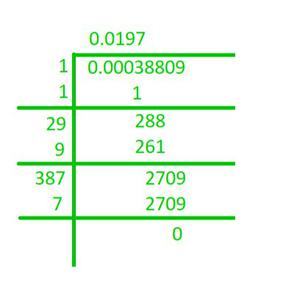Hence, the square root of 84.8241 is 9.21.

### Question 13) What is that fraction which when multiplied by itself gives 227.798629?

Solution:

We have to find the square root of the given number :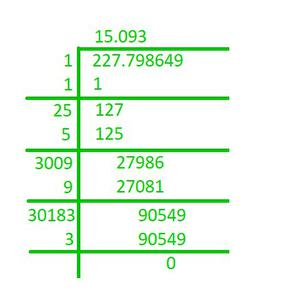Hence, the fraction, which when multiplied by itself, gives 227.798649 is 15.093.

### Question 14) The area of square playground is 256.6404 square meters. Find the length of one side of the playground.

Solution:

The length of one side of the playground is the square root of its area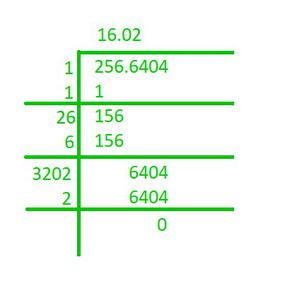So, the length of one side of the playground is 16.02 meters.

### Question 15) What is the fraction which when multiplied by itself gives 0.00053361?

Solution:

We have to find the square root of the given number :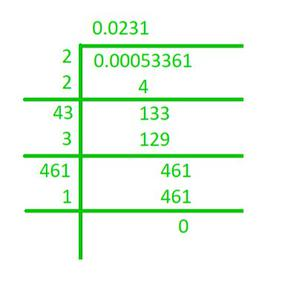Hence, the fraction, which when multiplied by itself, gives 0.00053361 is 0.0231.

### Question 16) Simplify:Solution:

1) First, finding the square roots of 5.29 and 59.29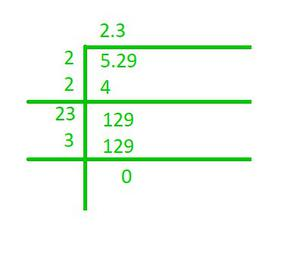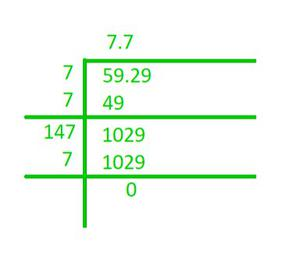On putting the values on their respective positions, we have (7.7 – 2.3) / (7.7 + 2.3) = 5.4/10 = 0.54.

2) First, finding the square roots of 0.2304 and 0.1764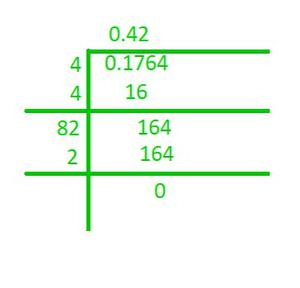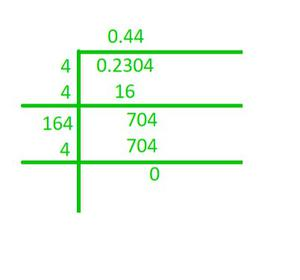On putting the values on their respective positions, we have (0.44 + 0.42) / (0.44 – 0.42) = 0.9/0.06 = 15.

### Question 17) Evaluate √50625 and hence find the value of √506.25 + √5.0625

Solution: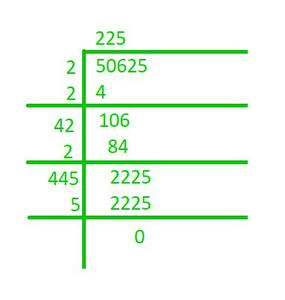Next, we calculate √506.25 and √5.0625

√506.25 = (√50625)/(√100) = 225/10 = 22.5

√5.0625 = (√50625)(√10000) = 225/100 = 2.25

√506.25 + √5.0625 = 22.5 + 2.25 = 24.75.

### Question 18) Find the value of √103.0225 and hence find the value of:

1) √10302.25          2) √1.030225

Solution:

First, finding the square root of 103.0225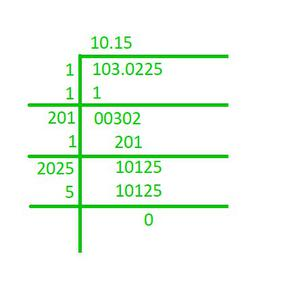Hence, the square root of 103.0225 is 10.15

Now, we can solve the following questions as shown below:

1) √10302.25 = (√103.0225)×(√100) = 10.15×10 = 101.5

2) √1.030225 = (√103.0225)/(√100) = 10.15/10 = 1.015

My Personal Notes arrow_drop_up